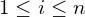# Shift-AND algorithm for exact pattern matching

The Shift-AND algorithm is similar to KMP and Z-algorithm, it inputs a pattern P and a long text T, outputs in linear time all occurrences of P in T. While KMP only maintains the longest prefix of P that is also a suffix of the examining prefix of T, Shift-AND keeps track of all prefixes of P that match any suffixes of the examining prefix of T. The algorithm is structured as below.

## Problem Input:

• a pattern P of length m.
• a text T of length n. Normally n is many times bigger than m.

As an example, we take:

• a pattern `P = "nina"` of length m = 4,
• and a text `T = "ninjaninan"` of length n = 10.

## Shift-AND:

### Initialization:

• create variable M (i.e. Mask): a dictionary of key-value pairs, where a key is a character of P and a value is a bitset of length m indicating the positions that the key appears in P. Note that for convenience, the order of the bitset is reversed (see below example).

`M = {"n": 0101, "i": 0010, "a": 1000}`

Notice how the order of every bitset is reversed, for example, while `"a"` appears as the last character of P, the first bit of `M["a"]` is set to 1. In general, if and only if a character C happens to be the i-th character of P, then the bit at position `m-i+1` of `M[C]` is set to 1.
• create variable A (i.e. Active): a bitset of length m indicating which prefixes of P match a suffix of the currently examining prefix of T. The bit order of A is also reversed, i.e. A`[i] == 1` if and only if the prefix of length `m-i+1` of P matches a suffix of the examining prefix of T. Initially, all bits in A are set to 0.

`A = 0000`

### Operation:

We iterate through each character of T, from position 1 to position n. At each step i (), we maintain the invariant of bitset A in time O(1):

• First, we shift A to the left by 1 (any match of length k in the previous step has the potential to become a match of length k+1 in this step).
• Then, the right-most bit of A is set to 1 (there might be a new match of length 1).
• Lastly, we apply bitwise `AND` to A and `M[the i-th character in T]` (for any match of length k in the previous step, it is extended to a match of length k+1 if and only if the k+1 -th character of P matches the i-th character of T). In short, at step i, we do

`A = [(A << 1) OR 1 ] AND M[i]`.

Step i=1:

Examining prefix of T: “n”

`A = [(A << 1) OR 1 ] AND M[i] = "0001" AND "0101" = "0001"`.

Only the last bit of A is 1, meaning only the length-1 prefix of P matches a suffix of the current prefix of T.

Step i=2:

Examining prefix of T: “ni”

`A = [(A OR 1)] AND M[i] = "0011" AND "0010" = "0010"`.

The second-to-last bit of A is 1, meaning the length-2 prefix of P matches a suffix of the current prefix of T.

Step i=3:

Examining prefix of T: “nin”

`A = [(A << 1) OR 1 ] AND M[i] = "0101" AND "0101" = "0101"`.

The length-1 and length-3 prefixes of P each matches a suffix of the current prefix of T.

Step i=4:

Examining prefix of T: “ninj”

`A = [(A << 1) OR 1 ] AND M[i] = "1011" AND "0000" = "0000"`.

Since the character `"j"` does not appear in P, its corresponding bitset defaults to all 0. At this step, no prefix of P matches any suffix of the current prefix of T.

Step i=9:

Examining prefix of T: “ninjanina”

`A = [(A << 1) OR 1 ] AND M[i] = "1011" AND "1000" = "1000"`.

At this step, since the first bit of A is set to 1, we have found an occurrence of P inside T.

Step i=10:

Examining prefix of T: “ninjaninan”

`A = [(A << 1) OR 1 ] AND M[i] = "0001" AND "0101" = "0001"`.

The last bit of A is 1, meaning the length-1 prefix of P matches a suffix of the current prefix of T.

Algorithm terminates.

## Output:

After each step, we check bitset A, if the first bit of A is 1 (implying that the prefix with length m of P matches a suffix of the current prefix of T) then output a match.

For this example, we found 1 occurrence of P in T (the matching ends at position 9 of T).

References:

• Shift-and visualization, Alvaro Videla, blog.

## One thought on “Shift-AND algorithm for exact pattern matching”

1.leakerlee says:

great